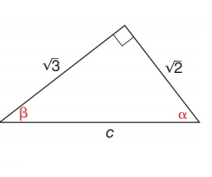Chapter 11.2, Problem 5EElementary Geometry For College St...

7th Edition
Alexander + 2 others
ISBN: 9781337614085

Solutions

Chapter
SectionElementary Geometry For College St...

7th Edition
Alexander + 2 others
ISBN: 9781337614085
Textbook Problem

In Exercises 1 to 6, find cos α and cos β .To determine

To find:

The cosα and cosβ.

Explanation

Consider the following figure,

General formula for cosine ratio is given below,

From the given figure the value c is the hypotenuse value since it is opposite to right angle.

Then 2 is the length of the leg adjacent to α and 3 is the length of leg adjacent to β.

The Pythagorean theorem is given below,

(hypotenuse)2=(lengthofoneleg)2+(lengthofotherleg)2

Substitute the values of hypotenuse=c,lengthofoneleg=3 andlengthofotherleg=2 to get the following,

Still sussing out bartleby?

Check out a sample textbook solution.

See a sample solution

The Solution to Your Study Problems

Bartleby provides explanations to thousands of textbook problems written by our experts, many with advanced degrees!

Get Started

84. If , find .

Mathematical Applications for the Management, Life, and Social Sciences

Change each decimal to a percent: 1.2

Elementary Technical Mathematics

A definite integral for the length of y = x3, 1 x 2 is a) 121+x3dx b) 121+x6dx c) 121+9x4dx d) 121+3x2dx

Study Guide for Stewart's Single Variable Calculus: Early Transcendentals, 8th